GOLD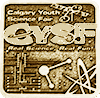#### Artificially Intelligent Agents for Bargaining With Private Information

This project uses deep reinforcement learning techniques to create a artificially intelligent agents for use in bargaining games involving private information analogous to real-life negotations scenarios.

## Introduction to game theoretic bargaining

In game theory, there are two main types of bargaining. First, bargaining with a shared asset pool. Assets is a resource that has value to agents within a game.  An agent is defined as a player of the game.  Each agent has a strategy, which is the decision-making process of the agent. A classic example of this first type of bargaining game is the splitting of some asset pool (a dollar or a cake), where each agents takes turns proposing a split of the asset pool, and the game ends whenever the opposing agent agrees to the division of assets 

This project is focused on the second type of bargaining, in which each agent already has a collection of different types and quantities of assets. This collection is called an asset portfolio. The general goal with this type of bargaining is for an agent to trade assets to increase the value of its own portfolio. To make this possible, there must be an imbalance in how the opposing agents value each type of asset. If each asset was valued equally by both agents, the game would be at a Nash equilibrium at the very beginning, in which there would be no trade that would be beneficial for both agents, and therefore both agents would be at an impasse . As explained in 1.3, this second type of bargaining is more common in the real-world, as parties in negotiations already have assets that they want to trade, rather than trying to divide an asset pool.

Reinforcement learning is a form of machine learning in which an agent learns to maximize a reward function by interacting with a given environment.  For example, an environment in which reinforcement learning could be applied would be game in which an agent must find an exit to the maze and avoid “enemies” wandering the maze. Every reinforcement learning algorithm has the same essential cyclical structure:

1. The agent chooses an action to perform based on a probability distribution of every possible action. Initially each action should be equally likely, and the agent should choose its action randomly. This is called the policy of the agent. In the example, an action would constitute going up, down, left, or right, if possible.
2. The environment updates itself, and returns the new environment new state, as well as a reward to the agent. In the example, most actions would return a reward of 0, except for when the agent exits the maze, or runs into an enemy, wherein the environment would return a reward of 1 and -1, respectively.
3. The agent updates itself to maximize the reward, based on the specific structure of the learning algorithm, whether it be Q-tables  or neural networks. In the example, the agent would update its policy  so that when the state of the environment is such that the agent is directly to the right of the agent, the probability that it would choose to go right in that state increases. Even if most actions return a reward of 0, once a non-zero reward is returned, the agent will have a memory of previous actions taken and
4. If the environment reaches an end-state, the environment restarts except that the agent has an updated policy. Otherwise, the environment continues with the updated state 

Over many iterations, the agent will be able to maximize the reward with every action it takes.

In bargaining environments, the environment has multiple agents, competing against each other. To effectively implement reinforcement learning in multiagent environments, a model of the opposing agents’ decisions has to be made. This can be achieved by having the agent playing against other reinforcement learning-based agents–.

There are many different algorithms that can be used to update the policy of the agent. Neural networks have shown themselves to be very computationally efficient as well as well performing in the context of reinforcement learning , . Neural networks take in a matrix of inputs, in this case, the state of the environment. The neural network then outputs the probability that each action should be taken. These are the base types of artificial neural networks (ANNs). In addition to ANNs, another possibility, especially in the context of bargaining, is to use recurrent neural networks (RNNs). These take very similar structure to neural networks, except that these RNNs can function more effectively with time-series data that changes length , such as a series of past bargaining decisions.

## Real-world bargaining scenarios

There are multiple real-world scenarios where automated bargaining can be implemented. In this project, there will be a focus on two main fields: real estate and merger negotiations. Human bargaining is complex and often an optimal solution is not obvious . Neural networks have already proven themselves to be more effective than humans in many reinforcement learning-based tasks including many games,  and voice recognition. Automated agents that either assist humans or completely replace humans in bargaining scenarios can be helpful, both in increasing the efficiency and efficacy of the bargaining process.

In real estate contracts, negotiations are based on both the price of the house as well as the terms of the sale, such as the completion date, arrangements to fix defects, and included appliances.  These specific terms of the sale can be modelled to function as assets in a bargaining game, and thus agents can learn how to effectively negotiate a real estate contract.

Within a merger or acquisition, negotiations take place based on the price of the merger or acquisition, as well as the combination of assets being acquired, and the method through which payment is made, usually through a combination of cash payment and common stock exchange. Mergers and acquisitions can also be modelled into by a bargaining game.  The considerations can be modelled as the assets in the bargaining game. These are two of many real-world bargaining scenarios that can be conceptualized as game theoretic bargaining games.

## Problem

With all of this given, the final goal of this project is to create a competent artificially intelligent agent in realistic bargaining games. The bargaining game described in this project is very similar to many real-world bargaining scenarios, such as real estate contracts and corporation mergers. Often, bargaining and negotiations in these scenarios are long, costly and can settle at unideal solutions due to human error. An automatic bargaining agent based on deep reinforcement learning can make the negotiation process in these scenarios more efficacious and efficient, reducing costs, time, as well as getting a more optimal settlement.

## Virtual Environment and Bargaining Game

The bargaining game is designed to be simple to train agents, allow for repeatability, and evaluate agent performance relative to other agents. A game creates a scenario between two agents, each of which has a private asset portfolio and a private preference vector. This game design allows for the value of asset types to either be independent or not. Over a fixed number of turns, the agents offer and counteroffer to try to maximize their own portfolio value. After the fixed number of turns, a second round is played with the roles reversed to impartially rank the two agents. A game goes through four stages:

Initial set-up: A number of asset types are created. Each agent participating in the game (agent 1 and agent 2) get assigned a private preference vector. Each agent is allocated a quantity of each of the previous asset types. The quantities of assets in each agent’s asset portfolios are private information. Finally, each agent is given the number of turns each round of the game will last.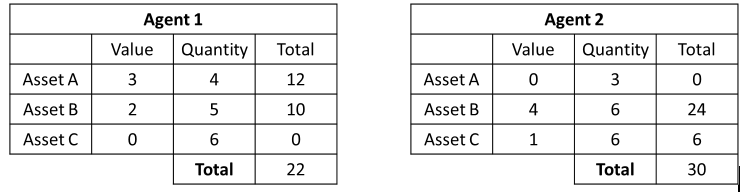Game progression: On every turn of the game, agent 1 generates an offer consisting of a vector of quantities of asset types to exchange for another vector of quantities of asset types from agent 2. Agent 2 will then accept or reject the offer. If agent 2 accepts, then the exchange is made and the agent portfolios are updated, otherwise the portfolios remain the same.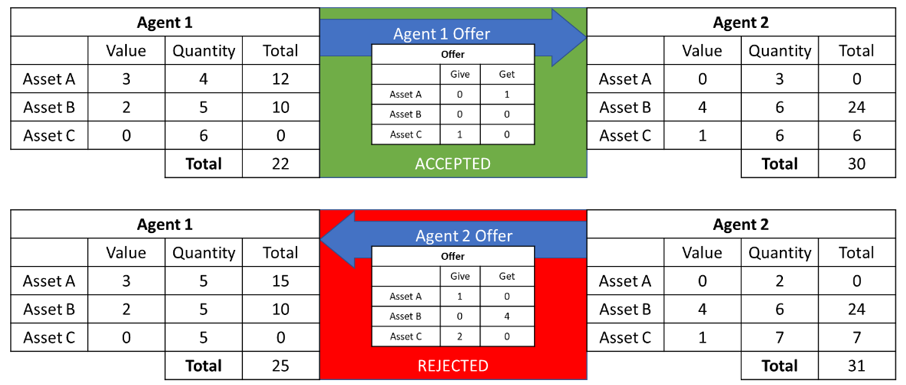Transition to round 2: After the fixed number of turns elapses, the value of each of the agents’ asset portfolios is calculated. The game is then replayed, with agent 2 taking the role of agent 1, and vice versa, in respect to their starting portfolios and preferences.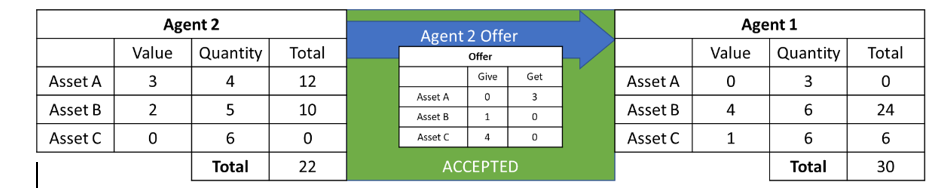End of game and evaluation of agents: Each agent is ranked based on the sum of the final asset portfolio values for each round. A higher sum would indicate the better performing agent in that game.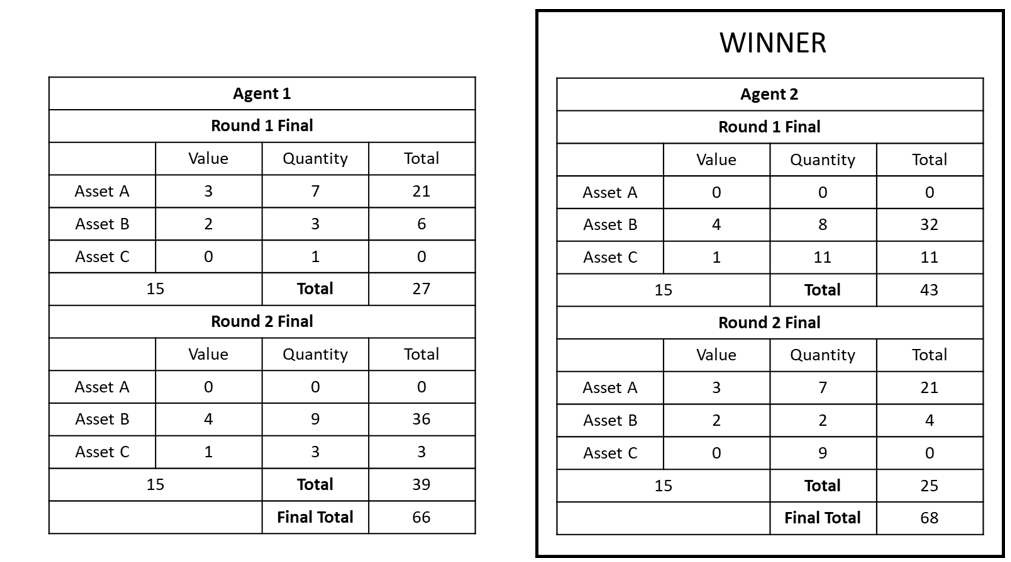In addition to the implementation of the bargaining game, the virtual environment communicates the responses of the bargaining game to the training algorithms of the agent and runs either a substantial number of simultaneous games or runs a single game with detailed history and description of the state of both the game and each agent.

## Agent evaluation in the bargaining game

The effectiveness of a single round of bargaining between two agents can either be determined by the comparative individual performance of each agent, or the combined performance measured by closeness to an optimum division of assets. The first evaluation method can simply be measured by comparing the value of each of the agents’ asset portfolio values by the end of the bargaining game and finding the extent of an agent’s effectiveness as a bargainer in comparison to the other agent by determining the difference between the two agent’s values. This metric measures only the relative performance between each agent participating in the bargaining scenario, and as such, if this method is used to measure the performances of four agents in two different bargaining scenarios, the agents in the separate bargaining scenarios cannot be compared across scenarios.

The second method of evaluation is a measure of how close both agents in a bargaining scenario are able to get to some theoretical optimal division of assets. If assets are able to be distributed in any way among the two agents, then the theoretical maximum asset portfolio value of both agents is giving all of one type of asset to the agent that prefers that type of asset the most. For example, an optimal division of asset, regardless of initial conditions would be: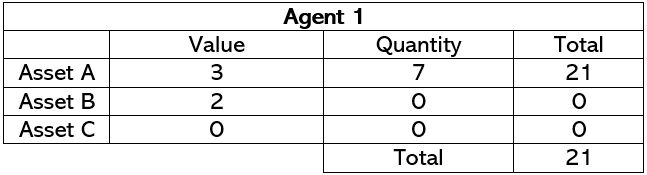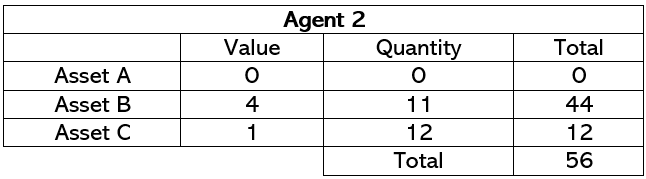As the sum of both agent’s portfolio values is the maximum possible sum, 77, given the initial quantities of available assets and the initial preferences of each agent, since each all of each asset type is given to the agent that prefers that asset type more. A simple way to measure how the agents performed is to measure the difference between the final sum of both of the agent’s asset portfolios and the optimal sum of the initial conditions. However, this theoretical optimum is overly simplistic, as it can oftentimes not be realistically achieved, because one agent will have to reduce its portfolio value in order to get to the optimum, as Agent 1 did in the example above.

A more realistic way of computing this optimum would be to find the highest possible sum of portfolio assets that would realistically be achieved, or in which the agents would not reduce their initial portfolio values. In the example, the adjusted optimum division would be: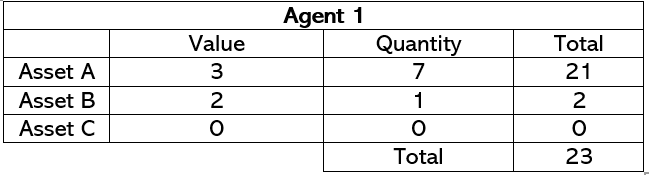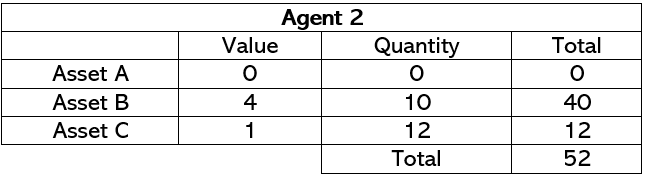This is because both agents have portfolio values above their initial portfolio values. The adjusted optimum summed portfolio value is 65.

1. ## Agent creation and training

2. The strategies and decision-making processes of each agent was mostly modelled with artificial neural networks. Different types and sizes of neural networks were employed and evaluated against eachother:
1. A single layered neural network, otherwise called a perceptron,
2. A shallow 4-layer neural network,
3. A deep 16-layer neural network,
4. A shallow 4-layer autoencoder, which is a normal artificial neural network with the exception that one of the intermediate layers containes a bottleneck of 4 nodes, rather than the normally hundred node layers.

Each neural network-based agent was trained using reinforcement learning over the course of multiple games. The reward that agents are trying to maximize is the final sum of the final asset portfolio values. During training, each agent plays against each other agent model, as well as a hardcoded agent, which makes actions based on a simple hardcoded ruleset, making the reinforcement learning both simultaneous and increasingly effective as each agent becomes better, making them better challenges for each other neural network. Each neural network was trained over a controlled number of iterations of a reinforcement learning cycle: 20000 iterations.

The ruleset that the simple hard-coded agent used during the reinforcement learning cycle was essentially making moves within the limitation that each proposal needed to increase it's personal asset portfolio value, and that it could only accept proposals that would increase it's personal asset portfolio value.

The framework used in this project was called PyTorch, an open-source Python library for machine learning. The other major machine learning library often used in machine learning research projects is TensorFlow, another python-based open-source library.  The main difference between these two frameworks are that TensorFlow is designed with industry applications in mind, with many of its capabilities focused on pipeline and web embedding implementations, while PyTorch is a more dynamic framework with less overhead and initializations needed to create usable models. Due to the need for the models used in this project to be integrated with the implementation of the bargaining game, the dynamic nature of PyTorch was preferred.

## Results

Results were evaluated for six different agents. The first two agents are non-neural networks:

• The simple hard-coded agent was an agent that was given a simple ruleset - only accept proposals that increase total asset portfolio value and only give proposals that would increase total asset portfolio value, but would otherwise make random actions. This agent was meant to simulate a simple real-world bargaining strategy, and would be the agent that neural networks would aim to perform better than.
• The random agent was an agent that made completely random actions - it would randomly accept or reject any proposal given to it, and would randomly make any valid proposal. This agent was meant to act as a negative control for the other agents, and it was expected that every other agent would perform better than the random agent.

The other 4 agents were all different configurations of neural networks trained through deep reinforcement learning:

• The single layer perceptron is a neural network structure with only a single layer between the input layer and the output/action layer.
• The shallow neural network is a neural network structure with 4 intermediate layers.
• The deep neural network is a neural network structure with 16 intermediate layers.
• The autoencoder network is similar to the shallow neural network in that it has 4 layers, however one of the intermediate layers has a bottleneck of nodes. Usually the intermediate layers of neural networks have hundreds of layers, but the bottleneck intermediate layer of the autoencoder only has 4 nodes. The reasoning behind this bottleneck is increasing the efficiency of training the autoencoder, due to only having to train 4 parameters for that layer rather than hundreds.

In order to evaluate the agents, a round robin system was established, wherein every agent model was tested against each other agent model. Two individual agent models going against eachother were evaluated by playing 100 rounds of the bargaining game against each other, and then taking the average final total asset portfolio value of each agent.

Table 1: Round-robin data of the average final total asset portfolio value for each agent against each other agent, including total performance as an average of all performances against all other agents.Additionally, in order to further evaluate the agents, each agent was again played against each other agent 1000 times with exactly the same initial conditions in each game. The final total summative asset portfolio value for both agents was then found

Table 2: Round-robin data of the average final total summative asset portfolio values for both agents combined in each matchup, with constructed 95% confidence intervals.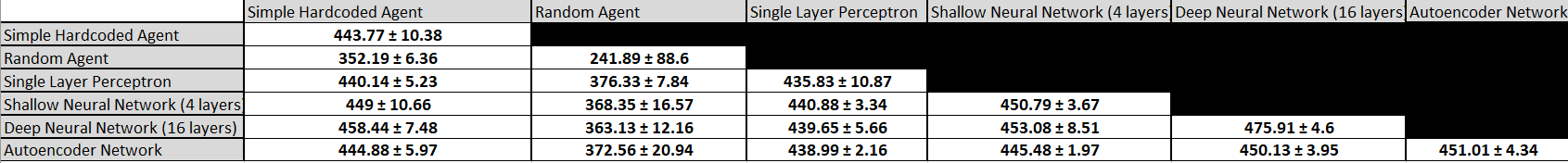## Analysis and Discussion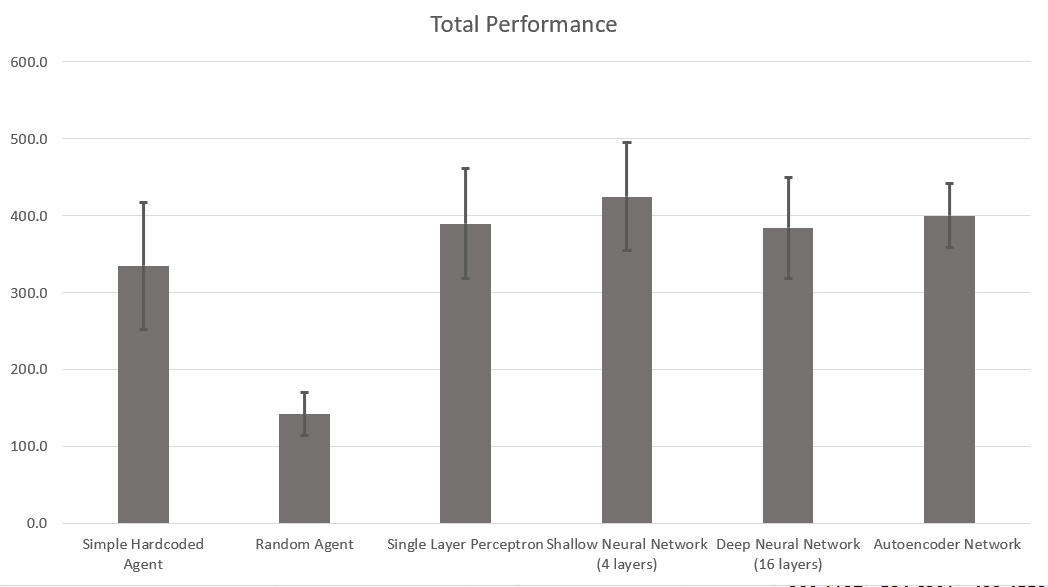In this graph, the total average performances of each agent over 100 games is shown. Because of the first evaluation method, each score by itself does not have any significance, and only by the relative performance compared to each other model can a model be evaluated as a more effective bargainer than any other given model. In this evaluation test, the best performing agents were the shallow neural network and the autoencoder, respectively, with the shallow neural network performing 24.6% better than my hardcoded agent and the autoencoder performing 18.4% better than the hardcoded agent. However, due to the size of the 95% confidence interval, each model's relative performance overlaps with each other model's relative performance except for the negative control model of the random agent, and thus very little conclusive statements about the relative performance of each model can be made.

In order to more clearly distinguish the performance of each agent with the first method of evaluation, the average difference between the final portfolio values was taken, in order to find average total differences and to calculate 95% confidence intervals. The scores shown in these tables are the differential performances, and can be negative. In a singular matchup, one agent's differential performance is the negative of the other agent's differential performance. Additionally, in order to reduce the size of the confidence intervals, the agents were played against each other for 1000 games for each matchup, rather than the previous 100 games.

Table 3:  Round-robin data of the average differential final total asset portfolio value for each agent against each other agent, including total differential performance as an average of all performances against all other agents played over a 1000 games.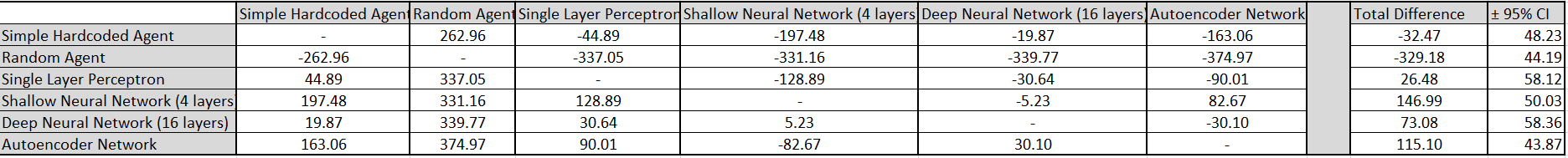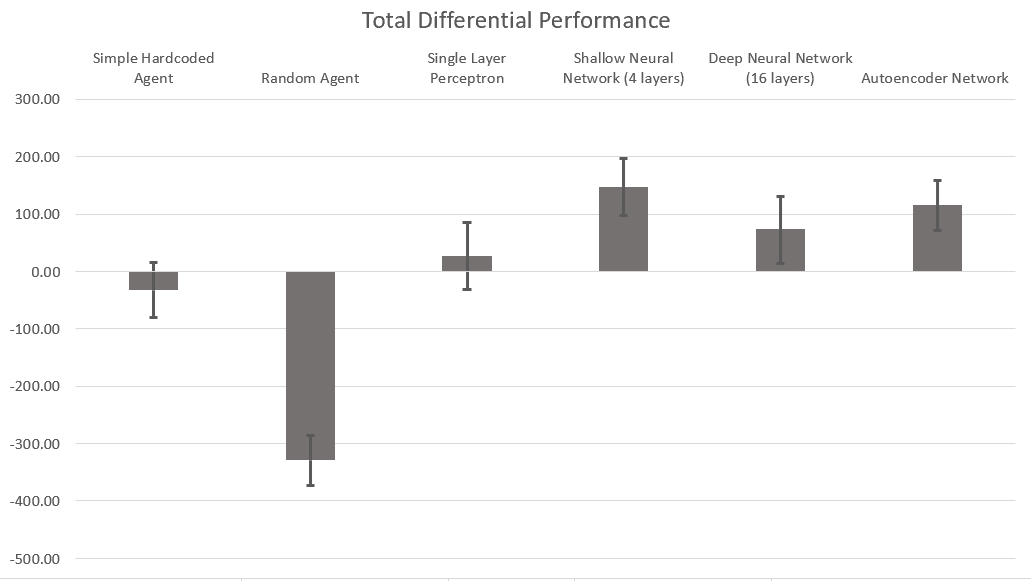In this graph, the shallow neural network and the autoencoder are again the relatively best performing agents, with average differential performances of 146.99 ± 50.03 and 115.10 ± 43.87, respectively. Each machine learning, created model had a positive differential performance, with only the simple hardcoded agent and the random agent (negative control) having negative differential performances.

Additionally, due to the reduced size of the 95% confidence intervals, the performance of the shallow neural network and the autoencoder can be deemed significantly better than the simple hardcoded agent, the human-emulated agent they were trained to beat, since the confidence interval do not overlap at all.

Due to the number of iterations each agent being controlled at 20000, a potential rationale for the autoencoder and the shallow neural network performing better than the deep neural network is due to the deep neural network being more inefficient in training, as there are exponentially more parameters needed to be properly learned for the deep neural network. If the amount of iterations the models were trained over was increased, the deep neural network has the potential to increase its overall performance.

With the first, relative method of evaluation, it was found that the shallow neural network was the best performing agent, being better than the simple hardcoded agent meant to emulate a human agent by a statistically significant margin.

In the second method of evaluation, each agent was trained again for 20000 iterations, with the exception that the initial conditions of the game were exactly the same for each game. An adjusted optimum was found for these initial conditions of the game: 498. The reward given to the agents during the deep reinforcement learning process, in addition to being the individual value of the agent's asset portfolio, was summed with the inverse of the difference between the adjusted optimum and the current summed portfolio values of both agents. Because of this change in the rewarding of agents, the agents would be incentivized to get the total sum of both of their portfolio values closer to the adjusted optimum value.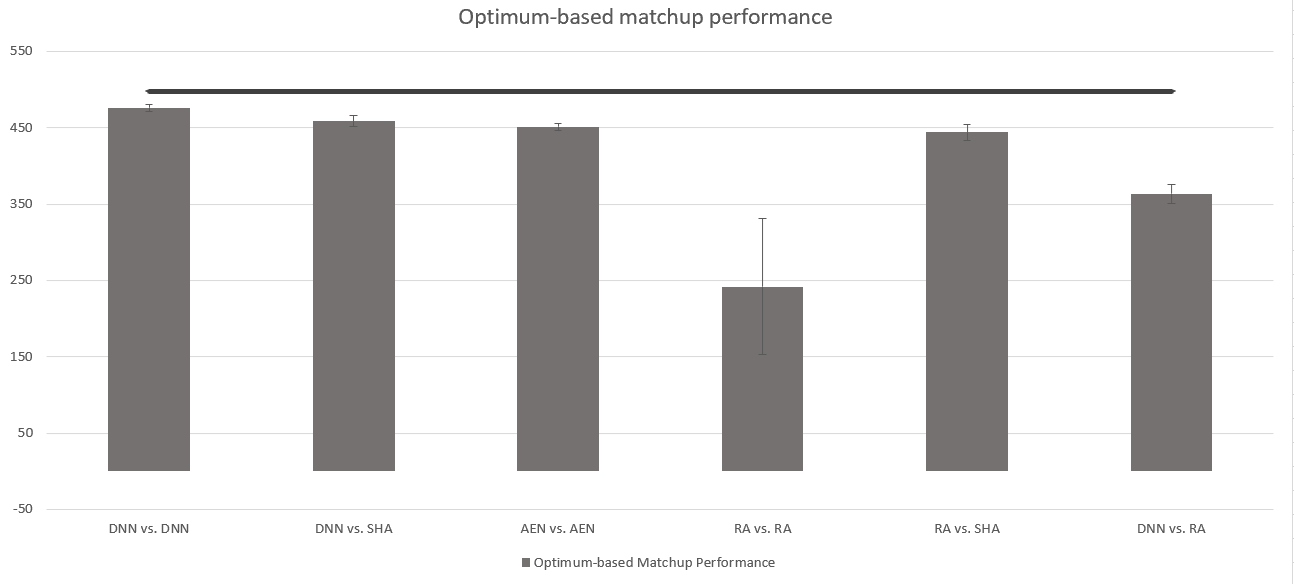Shown in this graph, the three matchups closest to the adjusted optimum performance of 498, and the three matchups farthest away from the adjusted optimum are shown.  As opposed to the previous method of evaluation, the matchup that performed the best with the second, optimum-based method of evaluation was the deep neural network playing against the deep neural network, with an average performance of 475.91 ± 4.60. This performance is statistically significantly better than any other matchup, with the closest being the deep neural network against the simple hardcoded agent with a score of 458.44 ± 7.48.

A potential explanation of why the deep neural network matchup performed best in this method of evalution rather than the first method of evaluation, where it performed comparatively worse to the shallow neural networks, is because of the increased complexity of the reward function. Rather than the increased amount of parameters in the deep neural network being a hindrance for the more simple first method of evaluation, in this second method of evaluation, the increased amount of parameters could have helped the agent better optimize itself to perform well with the new reward function than the reduced amount of parameters of the shallow neural network.

The confidence intervals for performances using machine learning models and the simple hardcoded agents are relatively narrow compared to the error bars involving random agents, as the trained agents converged to portfolio values relatively close to the adjusted optimum quickly during the evaluation performance, while the random agents, even if they do get portfolio values close to the adjusted optimum, can just as easily move away from that adjusted optimum.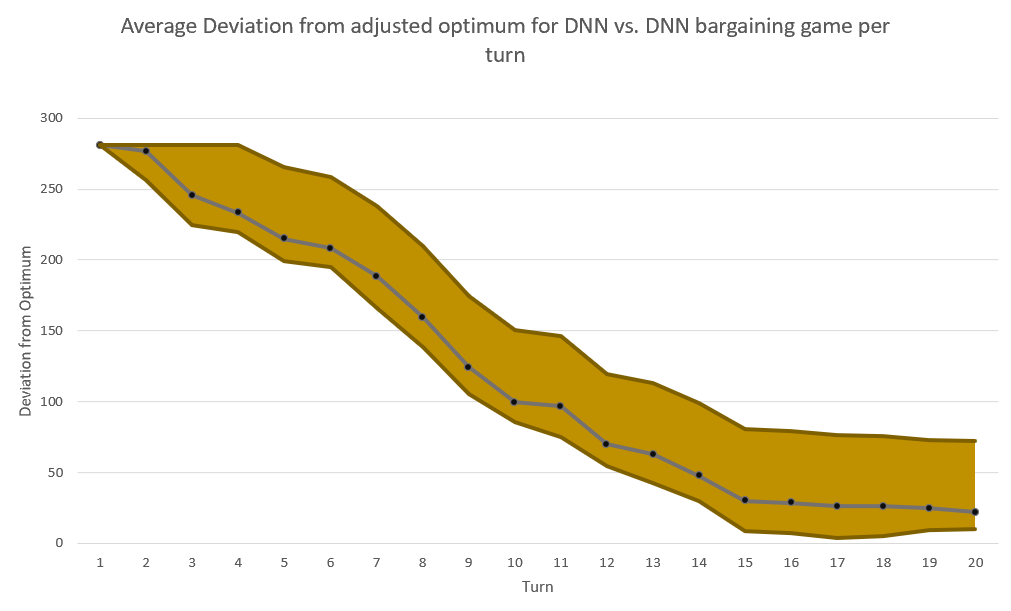Illustrated in this graph is the typical progression of a game between two deep neural networks during the evaluation process. Because initial conditions were equal, every single game started at the same summed portfolio value, 227, meaning that they also started at the same difference from the adjusted optimum: 271. The mustard-colored range shown in the graph is the interquartile range of the differences from the adjusted optimum over the progression of every turn in a 20 turn game. The actual line is the median difference between the adjusted optimum and the actual summed portfolio value between agents.

Notably, the difference from the adjusted optimum seems to plateau at approximately turn 15, meaning that the results of that games would not have been significantly different if the games went on for 16 turns or 20 turns. For most matchups, this trend is continued, with the summed portfolio values stagnating at around turn 15, implying that sigificant progress would not be made by adding a small amount (1-15) amount of turns to the game. It would be an interesting extension to see if changing the amount of turns in a game would change the agent's strategy, and cause a steeper drop in deviation from the adjusted optimum, or have the same results as shown above, except cut off at an earlier turn.

### Conclusion

The bargaining game described in this project is very similar to many real-world bargaining scenarios, such as real estate contracts and corporation mergers. Often, bargaining and negotiations in these scenarios are long, costly and can settle at unideal solutions due to human error. As shown in this project, an automatic bargaining agent based on deep reinforcement learning against already established bargaining scenarios can make the negotiation process in these scenarios more efficacious and efficient, reducing costs, time, and getting a more optimal settlement.

In the future, there are two major ways that this project can be improved. First, the variety of agent models can be expanded, in order to be able to experiment with different types of machine learning models as they can be applied to automatic bargaining. Specifically, recurrent neural networks and generative adversarial networks have proved themselves powerful in other contexts of deep reinforcement learning. Second, is to make the results of the project more applicable to previously expanded upon real-world bargaining and negotiation scenarios by a) changing the bargaining game to be more reflective of those scenarios and b) getting higher performing hard-coded strategies that are used in the real-world to use against machine learning agents in the deep reinforcement learning process.

Overall, this is a proof-of-concept project with an extremely high potential to be applied to various scenarios in the real world to greatly improve negotiation processes.

### Acknowledgement

Beatriz Garcia-Diaz, PhD from Webber Academy organizing the Applied Science course and constantly motivating me to succeed in my scientific pursuits.

Dhruv Mayank from ATB Financial and Raymond Patterson, PhD, MBA, CPA from UCalgary for giving criticisms and suggestions in the initial stages of my project, and helping me get a solid foundation to base the rest of my project on.

Alex Gierus, my father, for helping me tremendously every step of the way on this project, giving me guidance, advice and encouragement. He is the single most important reason that this project got started and made. Thank you so much!